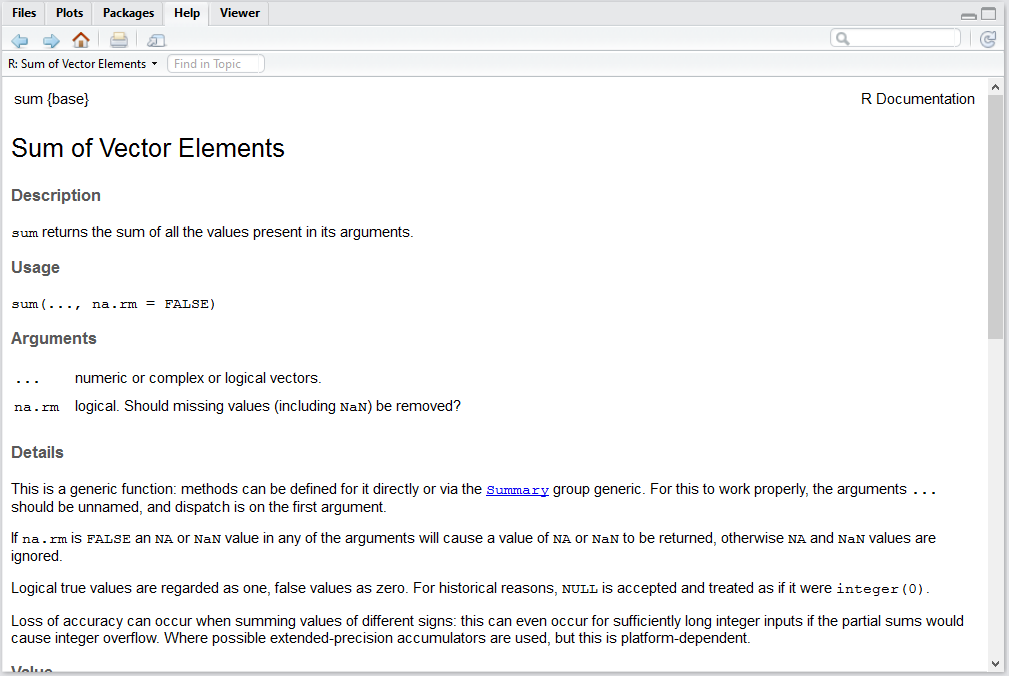## 2.4 Functions

Functions take in inputs, execute commands, and return output. They are extremely useful because if we have to make a particular calculation multiple times, we can just program a function and reuse it in the future. For example, sum is a function that takes in inputs of a numeric or integer vector, and returns the value of all the numbers added together.

### 2.4.1 Defining a function

The most basic function is a function that has does not return any value.

Hello<- function (...) {
cat("Hello World")
}
Hello()
## Hello World

Now, we define a function called myadd that adds 2 input numbers together and returns the total value.

myadd <- function (x, y) {
return(x+y)
}
myadd(3, 4)
##  7

From the example, we can see that a function consists of two parts: the 1st being the inputs, and the 2nd being the body of the function.

When you define a function, you need to specify how many inputs the function requires. How you name them is up to you, but it is likely you will need to use the names of the inputs in the body of the function. The body of the function is where you write the code for what you want the function to do with the inputs. Do not forget to include a return statement, otherwise there will be no output shown in the console for the function.

### 2.4.2 Vectorized operation

Since R can handle vector directly, our function can also take vectors as inputs:

myadd <- function (x, y) {
return(x+y)
}
a <- c(1,2,3)
b <- c(3,4,5)
myadd(a,b)
##  4 6 8

### 2.4.3 One-line function

Sometimes function can be very simple so that we can ignore the curly brackets:

f <- function(x) x^2+1
f(3)
##  10

### 2.4.4 Function with default input

We can also set default input value of a function:

myadd <- function (x, y=1) {
return(x+y)
}
myadd(3)
##  4
myadd(3, 4)
##  7

Now also that since R is a vectorized program, function can take vector as imputs:

myadd <- function (x, y) {
return(x+y)
}
myadd(1:3, 3:5)
##  4 6 8

### 2.4.5 Assignment of function outputs

You can also store the output of a function into another variable, by assigning the output to that variable.

For example, result stores the value of add(3,4), which is 7. When you call result, you will then get the value 7.

result <- myadd(3, 4)
result 
##  7

### 2.4.6 Help on function

Type a function’s name at the console, you can see the code behind. If you Type “? function’s name”, you will see its help file as in Figure 2.1.

? sumFigure 2.1: Help content for sum in RStudio

### 2.4.7 Source code of a function

If you type the function name without bracket, then it will show the code of the function.

myadd <- function (x, y) {
return(x+y)
}
myadd
## function (x, y) {
##        return(x+y)
## }